Скачать презентацию 1 LECTURE 27 IP ADDRESSING Binary

76f6a0968a8d9698532519a355902d76.ppt

• Количество слайдов: 44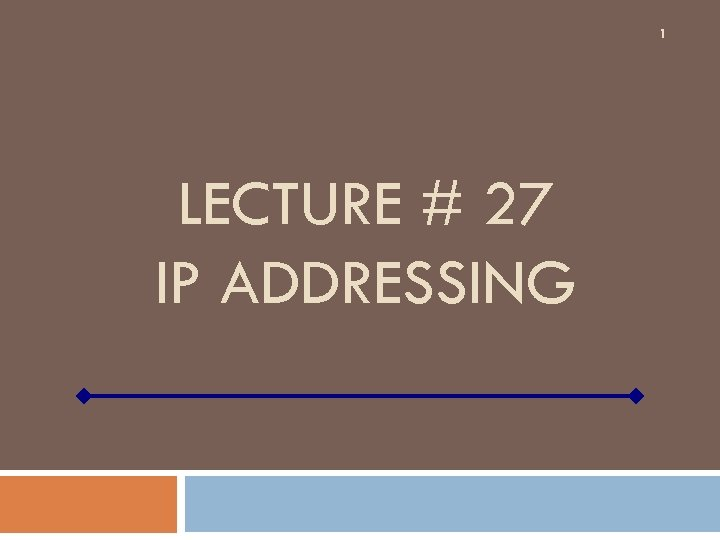1 LECTURE # 27 IP ADDRESSING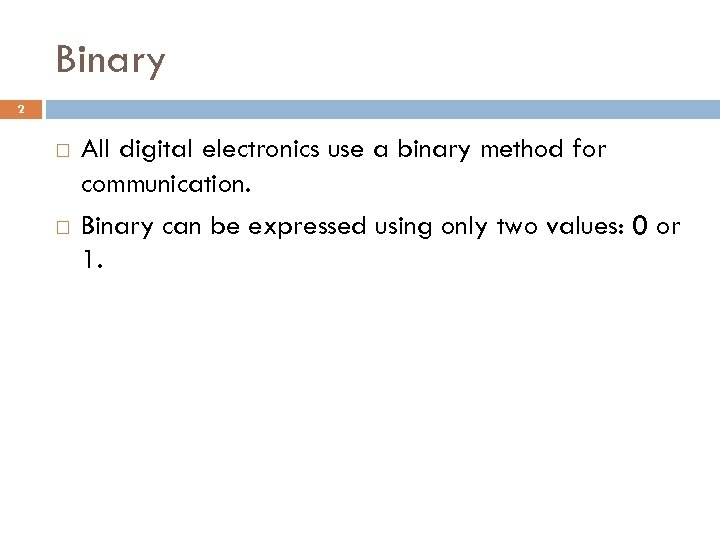Binary 2 All digital electronics use a binary method for communication. Binary can be expressed using only two values: 0 or 1.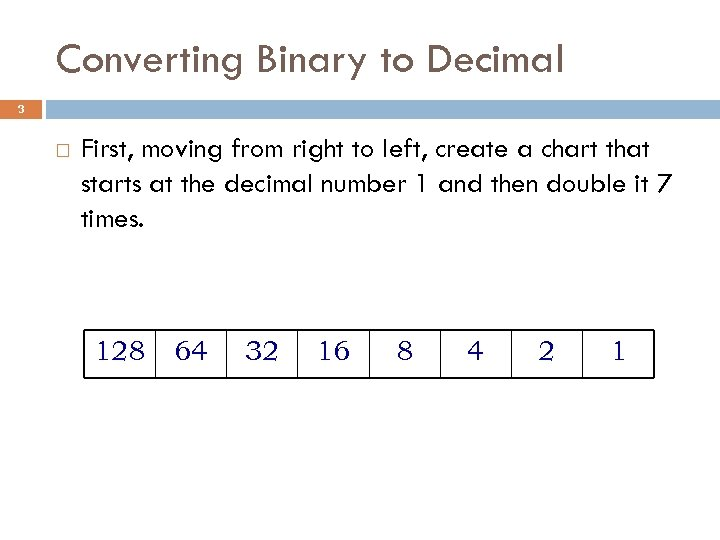Converting Binary to Decimal 3 First, moving from right to left, create a chart that starts at the decimal number 1 and then double it 7 times. 128 64 32 16 8 4 2 1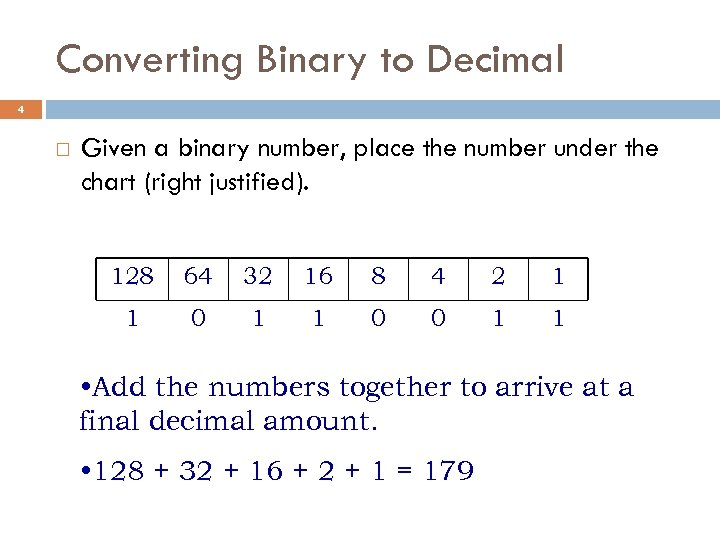Converting Binary to Decimal 4 Given a binary number, place the number under the chart (right justified). 128 64 32 16 8 4 2 1 1 0 0 1 1 • Add the numbers together to arrive at a final decimal amount. • 128 + 32 + 16 + 2 + 1 = 179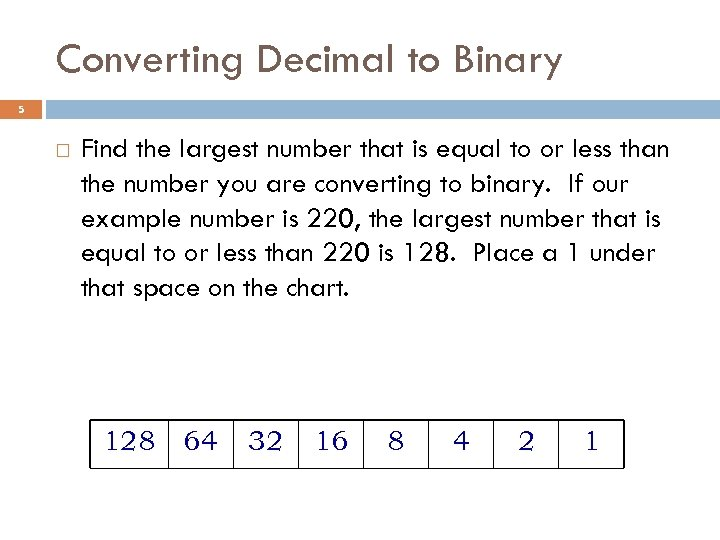Converting Decimal to Binary 5 Find the largest number that is equal to or less than the number you are converting to binary. If our example number is 220, the largest number that is equal to or less than 220 is 128. Place a 1 under that space on the chart. 128 64 32 16 8 4 2 1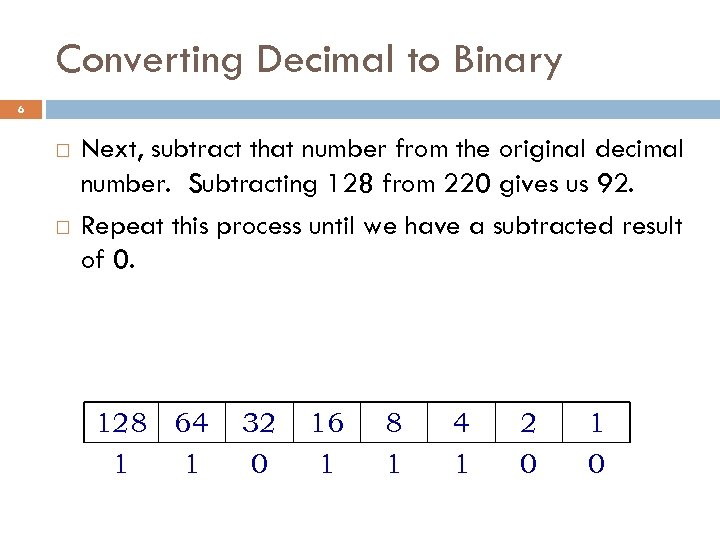Converting Decimal to Binary 6 Next, subtract that number from the original decimal number. Subtracting 128 from 220 gives us 92. Repeat this process until we have a subtracted result of 0. 128 64 1 1 32 0 16 1 8 1 4 1 2 0 1 0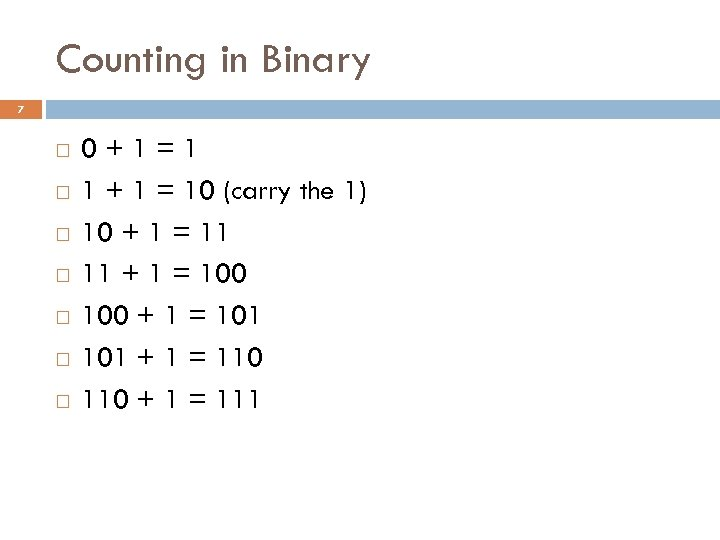Counting in Binary 7 0+1=1 1 + 1 = 10 (carry the 1) 10 + 1 = 11 11 + 1 = 100 + 1 = 101 + 1 = 110 + 1 = 111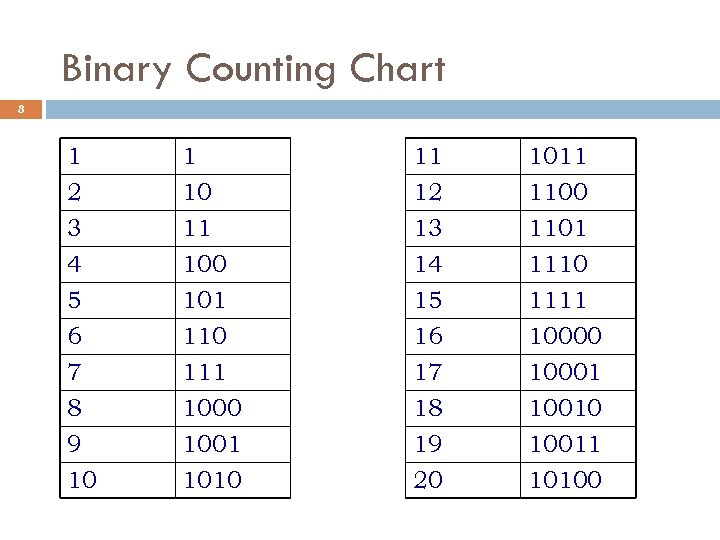Binary Counting Chart 8 1 2 3 4 1 10 11 100 11 12 13 14 1011 1100 1101 1110 5 6 7 8 9 10 101 110 111 1000 1001 1010 15 16 17 18 19 20 1111 10000 10001 10010 10011 10100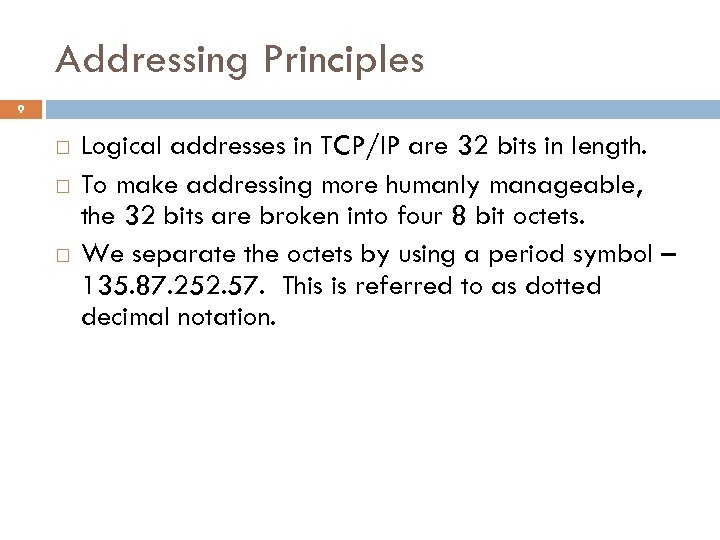Addressing Principles 9 Logical addresses in TCP/IP are 32 bits in length. To make addressing more humanly manageable, the 32 bits are broken into four 8 bit octets. We separate the octets by using a period symbol – 135. 87. 252. 57. This is referred to as dotted decimal notation.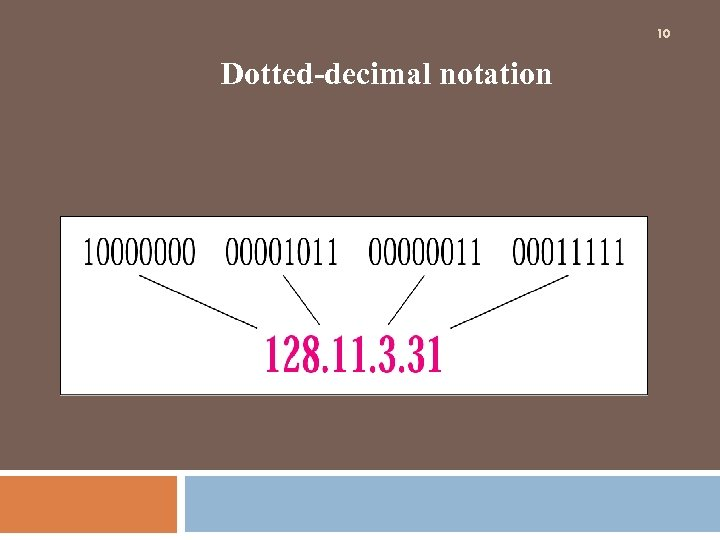10 Dotted-decimal notation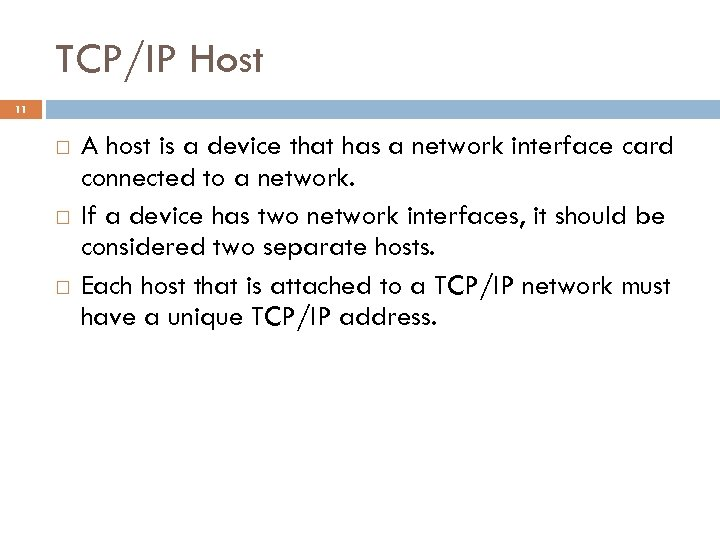TCP/IP Host 11 A host is a device that has a network interface card connected to a network. If a device has two network interfaces, it should be considered two separate hosts. Each host that is attached to a TCP/IP network must have a unique TCP/IP address.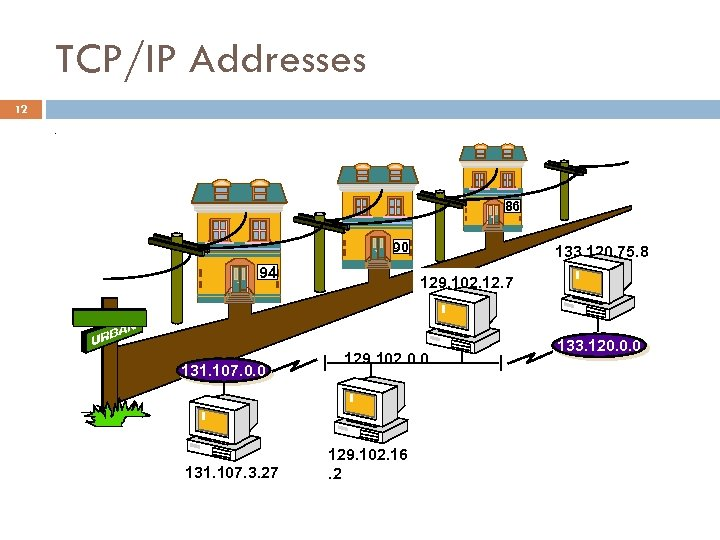TCP/IP Addresses 12. 86 90 94 133. 120. 75. 8 129. 102. 12. 7 MARIA AVE 131. 107. 0. 0 131. 107. 3. 27 129. 102. 0. 0 129. 102. 16. 2 133. 120. 0. 0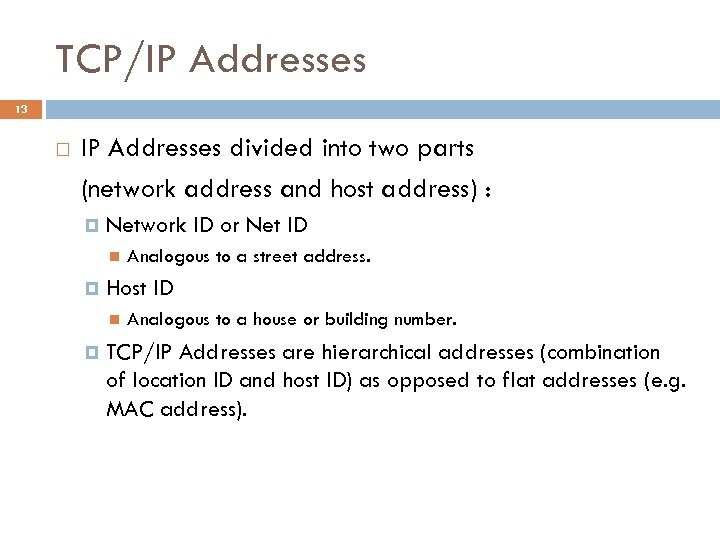TCP/IP Addresses 13 IP Addresses divided into two parts (network address and host address) : Network ID or Net ID Host ID Analogous to a street address. Analogous to a house or building number. TCP/IP Addresses are hierarchical addresses (combination of location ID and host ID) as opposed to flat addresses (e. g. MAC address).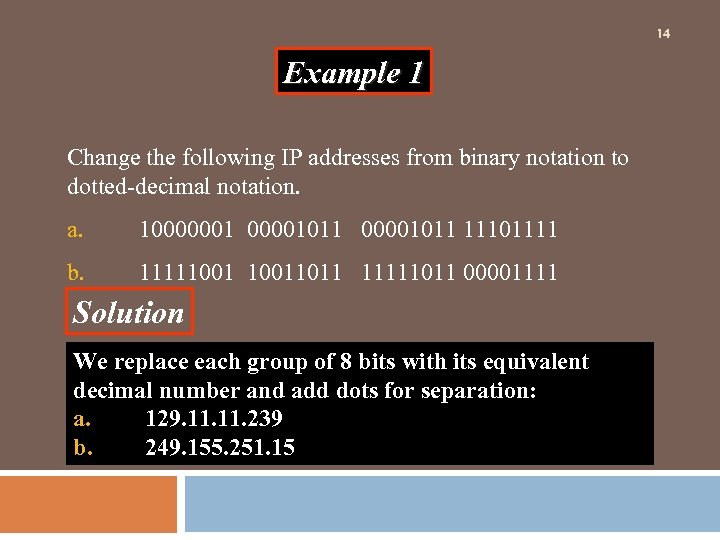14 Example 1 Change the following IP addresses from binary notation to dotted-decimal notation. a. 10000001011 11101111 b. 111110011011 11111011 00001111 Solution We replace each group of 8 bits with its equivalent decimal number and add dots for separation: a. 129. 11. 239 b. 249. 155. 251. 15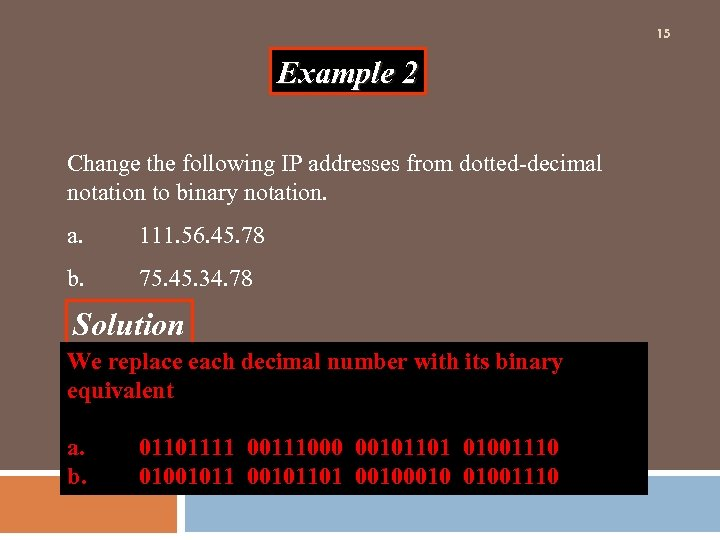15 Example 2 Change the following IP addresses from dotted-decimal notation to binary notation. a. 111. 56. 45. 78 b. 75. 45. 34. 78 Solution We replace each decimal number with its binary equivalent a. b. 01101111 00111000 00101101 01001110 0100101101 0010 01001110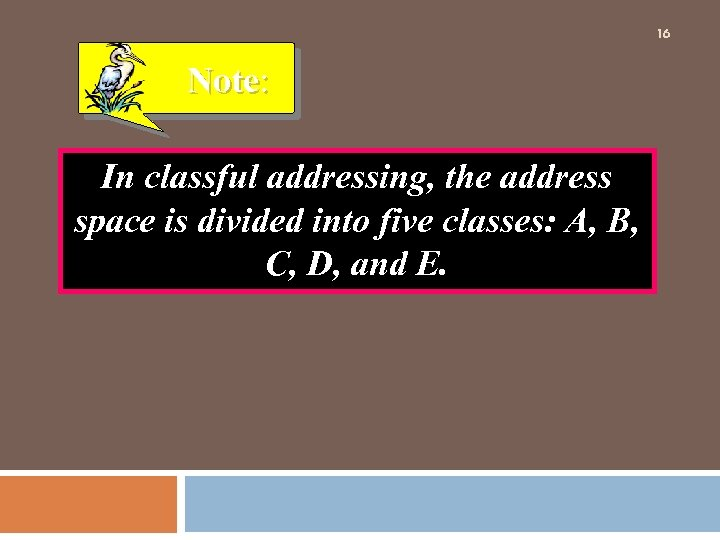16 Note: In classful addressing, the address space is divided into five classes: A, B, C, D, and E.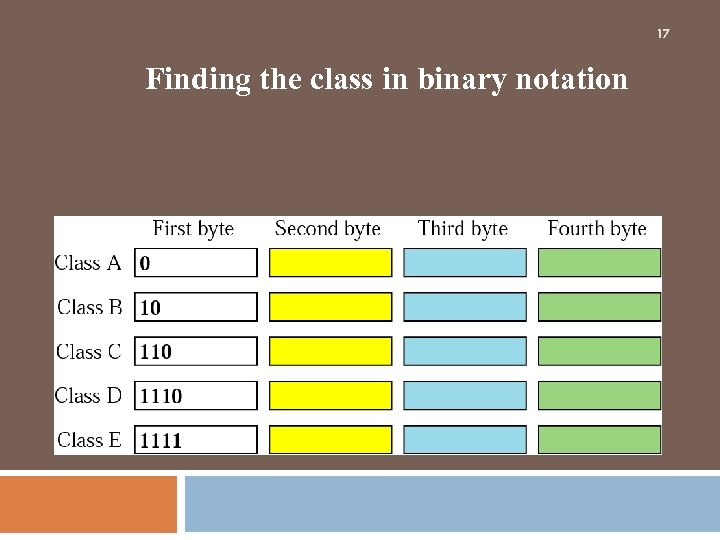17 Finding the class in binary notation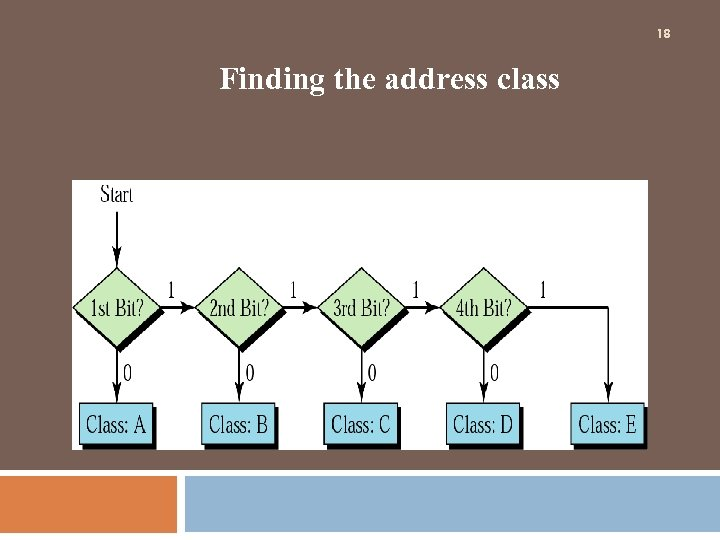18 Finding the address class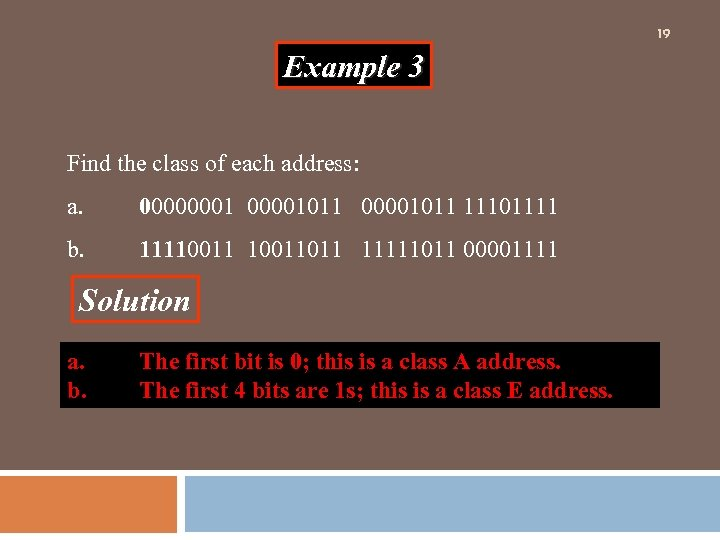19 Example 3 Find the class of each address: a. 00000001011 11101111 b. 11110011011 11111011 00001111 Solution a. b. The first bit is 0; this is a class A address. The first 4 bits are 1 s; this is a class E address.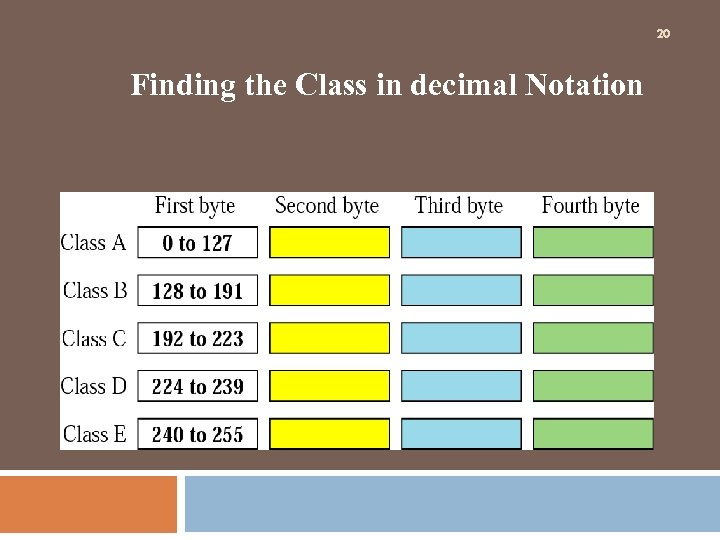20 Finding the Class in decimal Notation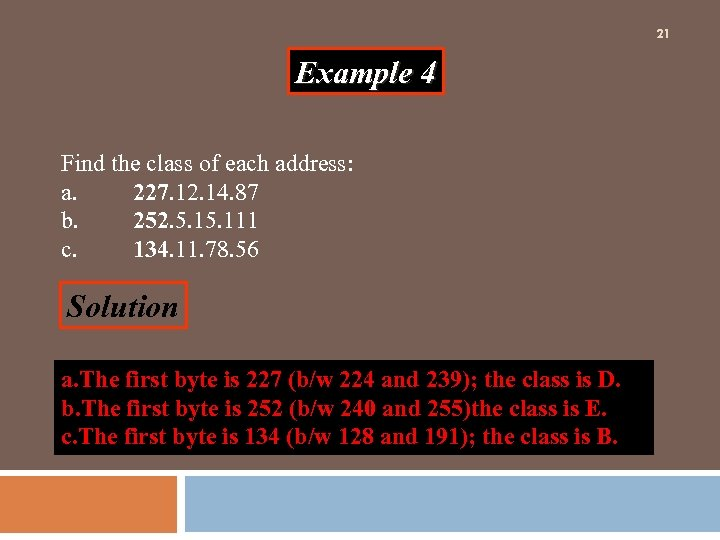21 Example 4 Find the class of each address: a. 227. 12. 14. 87 b. 252. 5. 111 c. 134. 11. 78. 56 Solution a. The first byte is 227 (b/w 224 and 239); the class is D. b. The first byte is 252 (b/w 240 and 255)the class is E. c. The first byte is 134 (b/w 128 and 191); the class is B.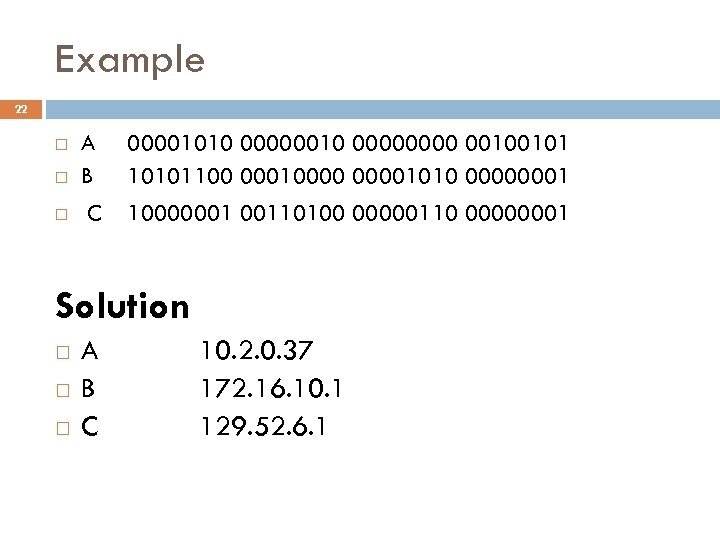Example 22 A B 00001010 00000000 00100101 10101100 000100001010 00000001 C 10000001 00110100 00000110 00000001 Solution A B C 10. 2. 0. 37 172. 16. 10. 1 129. 52. 6. 1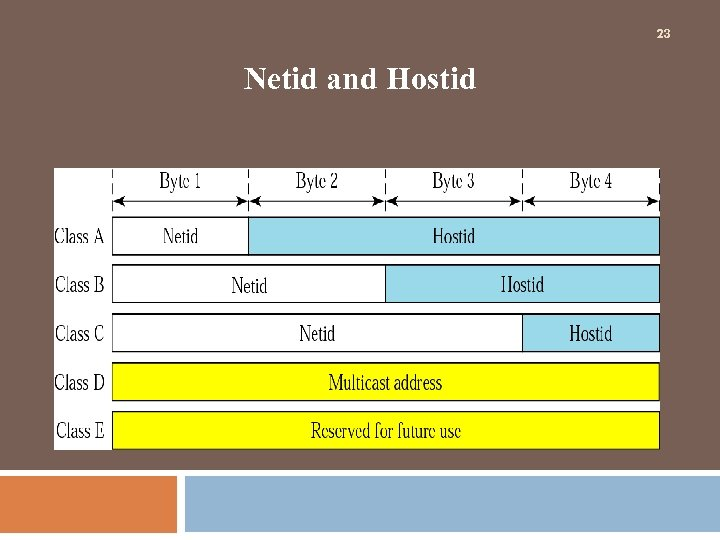23 Netid and Hostid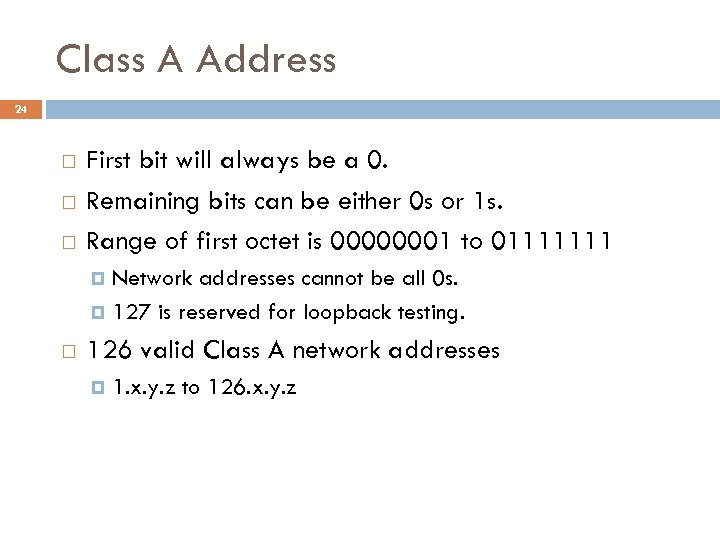Class A Address 24 First bit will always be a 0. Remaining bits can be either 0 s or 1 s. Range of first octet is 00000001 to 01111111 Network addresses cannot be all 0 s. 127 is reserved for loopback testing. 126 valid Class A network addresses 1. x. y. z to 126. x. y. z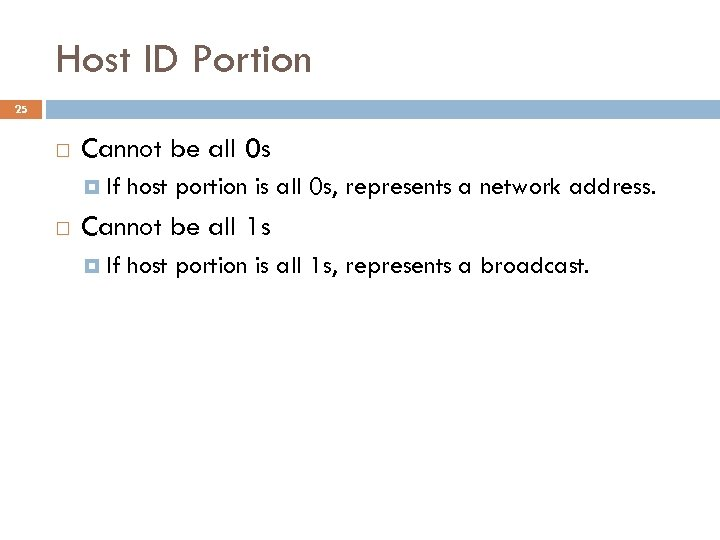Host ID Portion 25 Cannot be all 0 s If host portion is all 0 s, represents a network address. Cannot be all 1 s If host portion is all 1 s, represents a broadcast.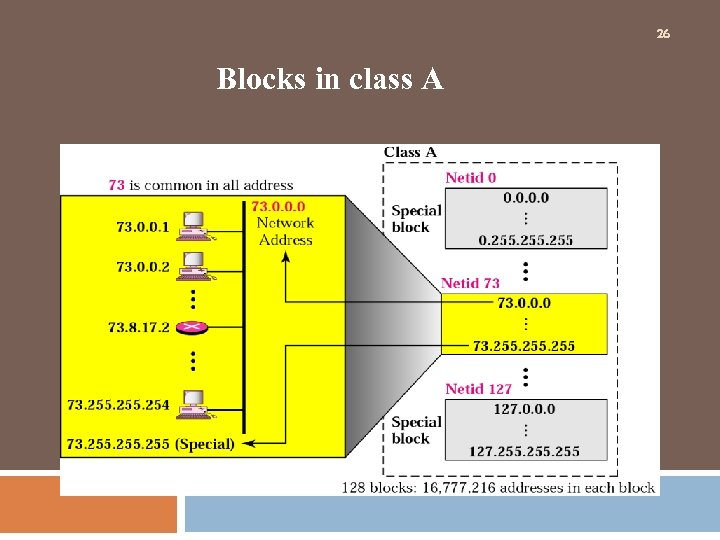26 Blocks in class A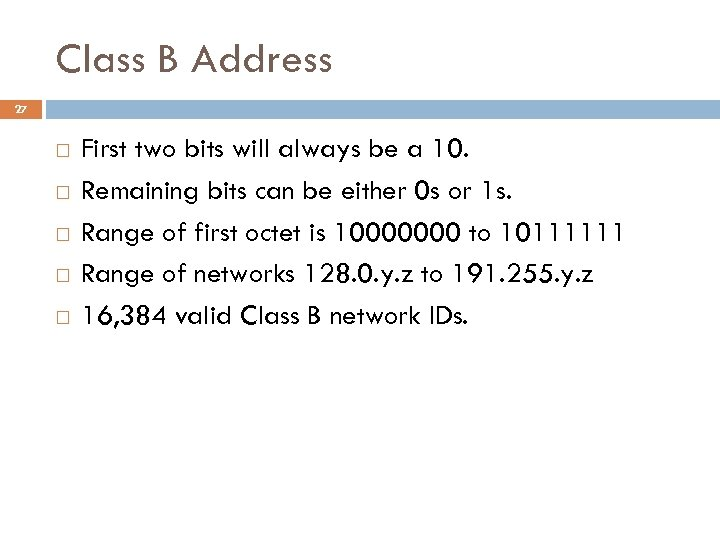Class B Address 27 First two bits will always be a 10. Remaining bits can be either 0 s or 1 s. Range of first octet is 10000000 to 10111111 Range of networks 128. 0. y. z to 191. 255. y. z 16, 384 valid Class B network IDs.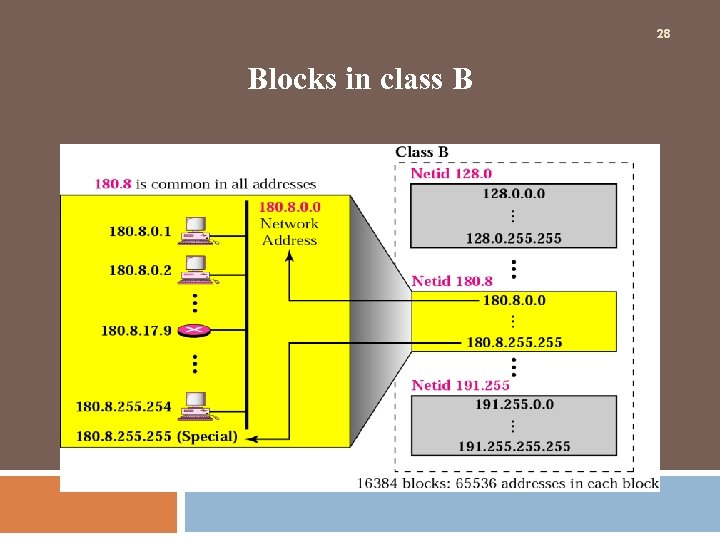28 Blocks in class B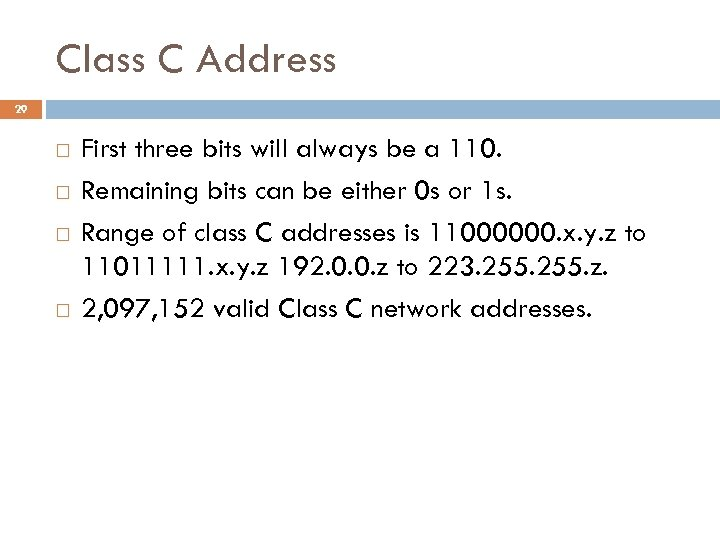Class C Address 29 First three bits will always be a 110. Remaining bits can be either 0 s or 1 s. Range of class C addresses is 11000000. x. y. z to 11011111. x. y. z 192. 0. 0. z to 223. 255. z. 2, 097, 152 valid Class C network addresses.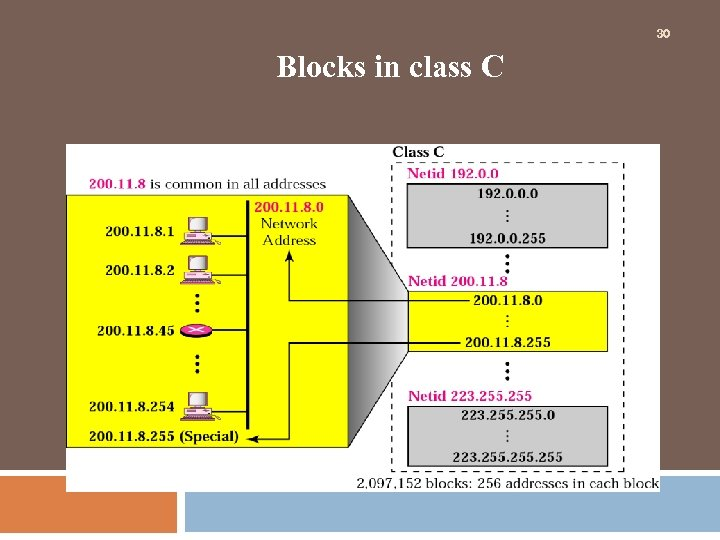30 Blocks in class C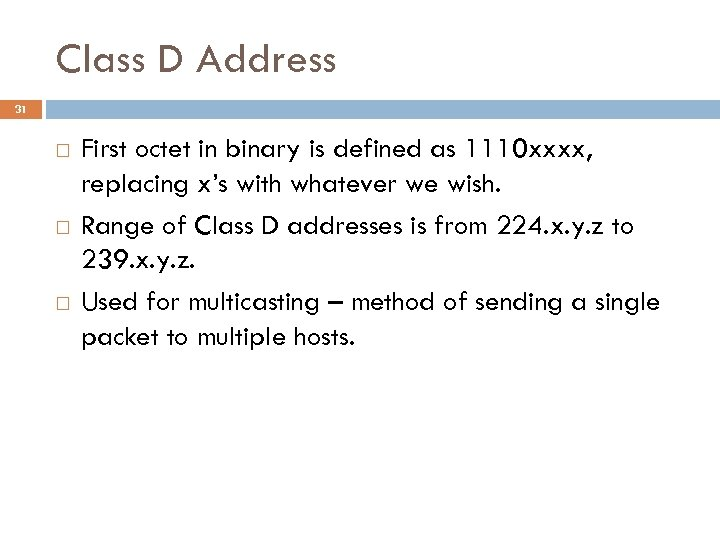Class D Address 31 First octet in binary is defined as 1110 xxxx, replacing x’s with whatever we wish. Range of Class D addresses is from 224. x. y. z to 239. x. y. z. Used for multicasting – method of sending a single packet to multiple hosts.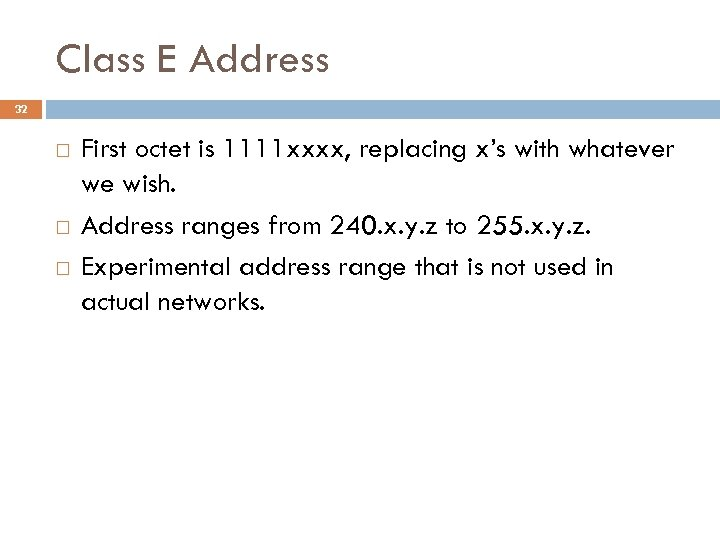Class E Address 32 First octet is 1111 xxxx, replacing x’s with whatever we wish. Address ranges from 240. x. y. z to 255. x. y. z. Experimental address range that is not used in actual networks.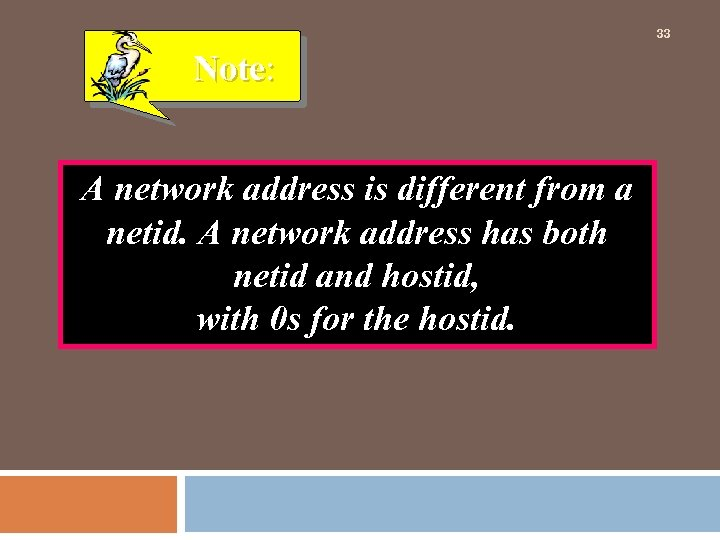33 Note: A network address is different from a netid. A network address has both netid and hostid, with 0 s for the hostid.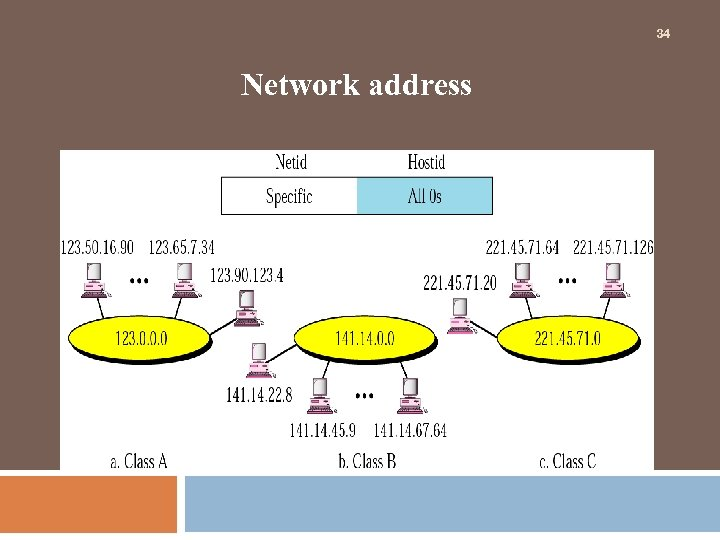34 Network address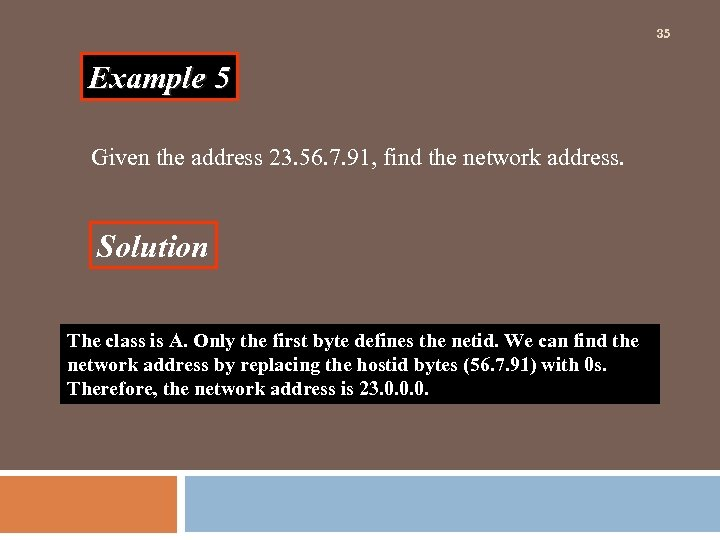35 Example 5 Given the address 23. 56. 7. 91, find the network address. Solution The class is A. Only the first byte defines the netid. We can find the network address by replacing the hostid bytes (56. 7. 91) with 0 s. Therefore, the network address is 23. 0. 0. 0.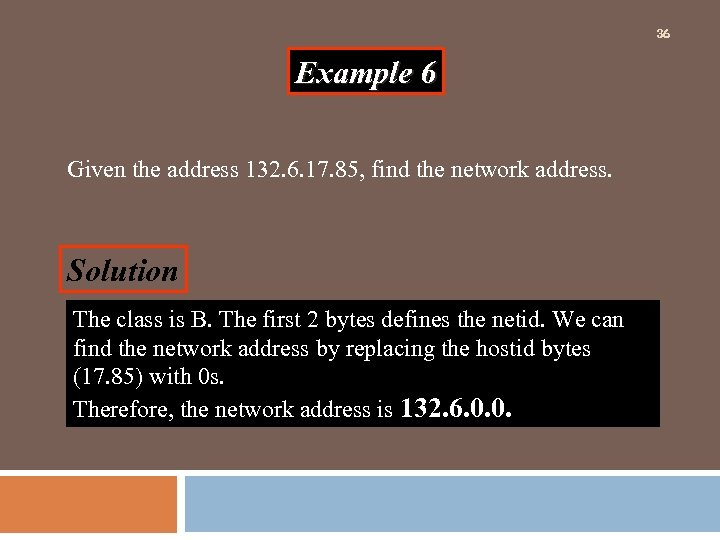36 Example 6 Given the address 132. 6. 17. 85, find the network address. Solution The class is B. The first 2 bytes defines the netid. We can find the network address by replacing the hostid bytes (17. 85) with 0 s. Therefore, the network address is 132. 6. 0. 0.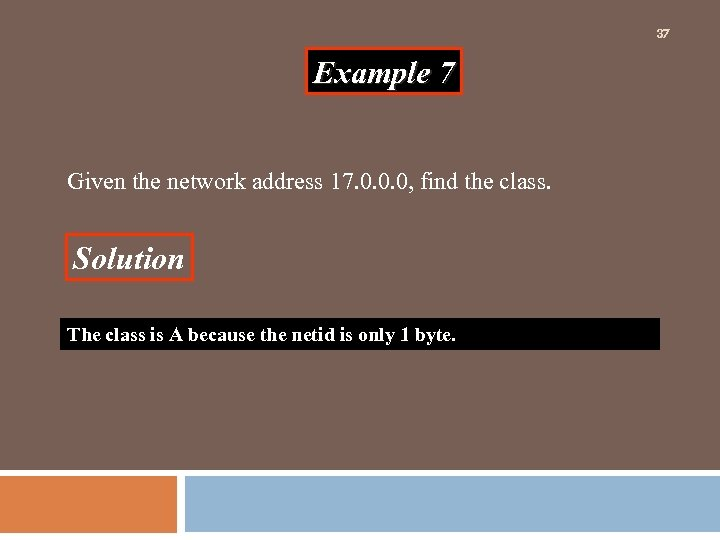37 Example 7 Given the network address 17. 0. 0. 0, find the class. Solution The class is A because the netid is only 1 byte.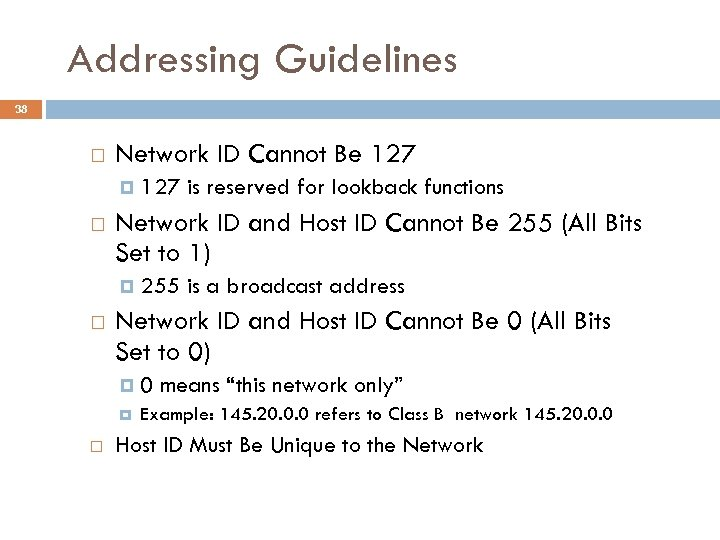Addressing Guidelines 38 Network ID Cannot Be 127 Network ID and Host ID Cannot Be 255 (All Bits Set to 1) 127 is reserved for lookback functions 255 is a broadcast address Network ID and Host ID Cannot Be 0 (All Bits Set to 0) 0 means “this network only” Example: 145. 20. 0. 0 refers to Class B network 145. 20. 0. 0 Host ID Must Be Unique to the Network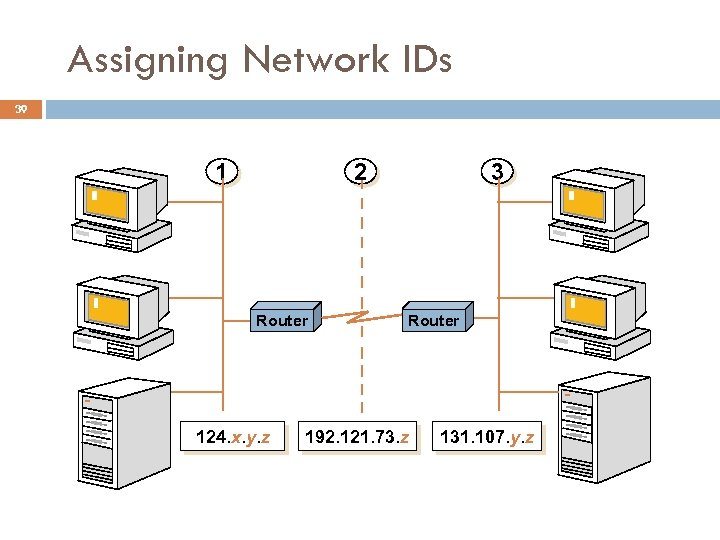Assigning Network IDs 39 1 2 Router 124. x. y. z 3 Router 192. 121. 73. z 131. 107. y. z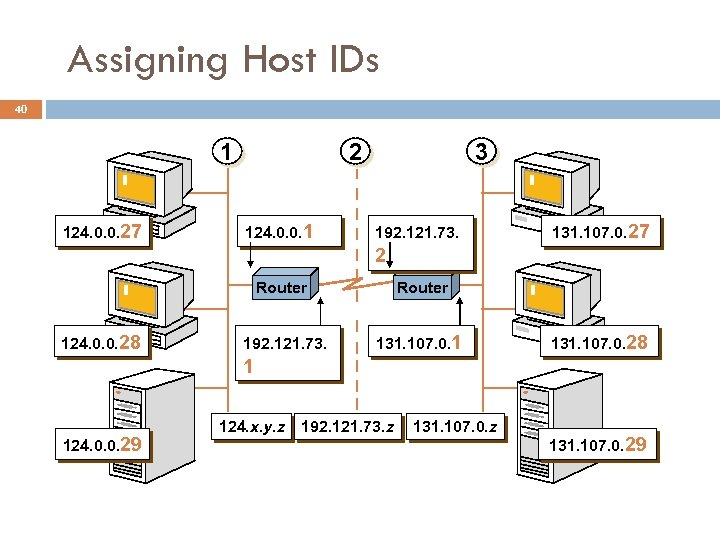Assigning Host IDs 40 1 124. 0. 0. 27 2 124. 0. 0. 1 3 192. 121. 73. 131. 107. 0. 27 2 Router 124. 0. 0. 28 Router 192. 121. 73. 131. 107. 0. 1 131. 107. 0. 28 1 124. 0. 0. 29 124. x. y. z 192. 121. 73. z 131. 107. 0. 29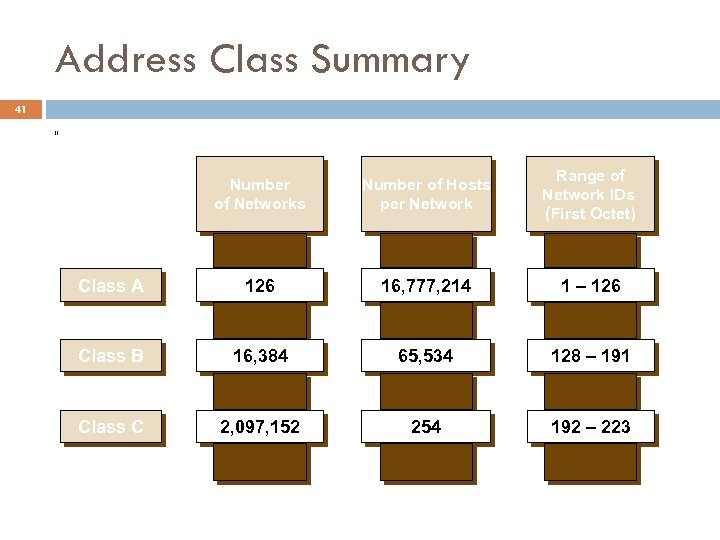Address Class Summary 41 [[ Number of Networks Number of Hosts per Network Range of Network IDs (First Octet) Class A 126 16, 777, 214 1 – 126 Class B 16, 384 65, 534 128 – 191 Class C 2, 097, 152 254 192 – 223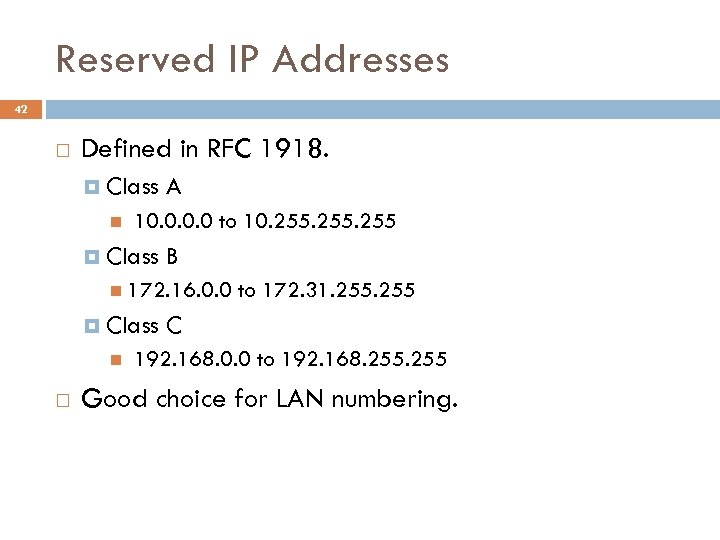Reserved IP Addresses 42 Defined in RFC 1918. Class A 10. 0 to 10. 255 Class B 172. 16. 0. 0 Class to 172. 31. 255 C 192. 168. 0. 0 to 192. 168. 255 Good choice for LAN numbering.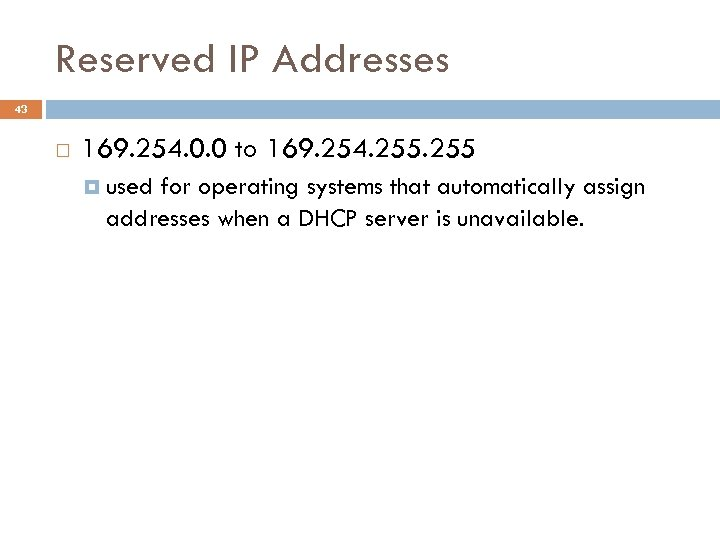Reserved IP Addresses 43 169. 254. 0. 0 to 169. 254. 255 used for operating systems that automatically assign addresses when a DHCP server is unavailable.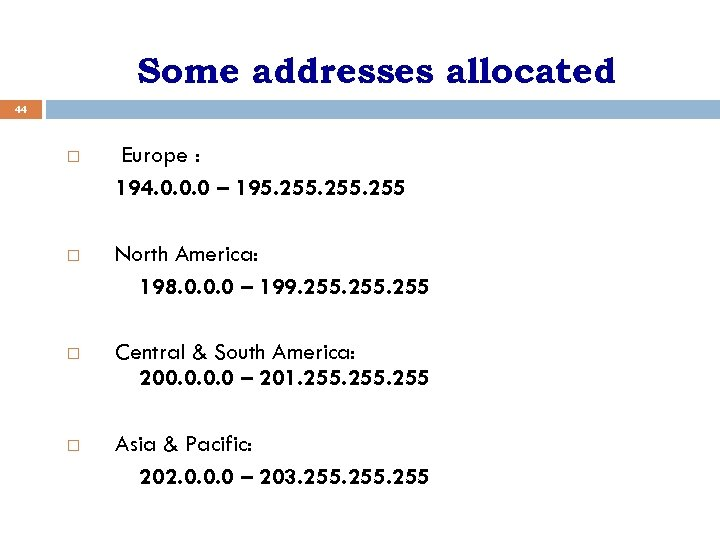Some addresses allocated 44 Europe : 194. 0. 0. 0 – 195. 255 North America: 198. 0. 0. 0 – 199. 255 Central & South America: 200. 0 – 201. 255 Asia & Pacific: 202. 0. 0. 0 – 203. 255